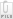Pay results fee

Sorry, you have not enough credits to pay results fee.

# What is the solution to the bowl & sphere paradox?

Launcher poly Australia
Status Closed Mediated Closed 2 years, 7 months ago
Bounty Reward
25

### Labels

paradoxes gravitation ; gravity

### Description

In this paradox, an open circular bowl of mass Mb has its centre of gravity (O) a distance r above the centre of its floor (see attachment Bowl&Sphere.jpg). A sphere of mass Ms and radius r is placed in the bowl. This sphere also has its centre of gravity at O.

According to gravitational laws, the gravitational force F between the sphere and the bowl is Mb*Ms/d^2, where d is the distance between their centres of gravity.

In the present case, both bowl and sphere have their centres of gravity at the point O, so d=0, and the gravitational force between the objects is infinite. Explain this. (ZBL#149).Bowl&Sphere.jpg

### Answers (2)

•Jun 27, 2012

The short and simple answer is that there is an assumption being made here that holds for one object but not the other. This assumption is that an object with a spherically-symmetric distribution of mass exerts the same gravitational attraction on external bodies as if all the sphere's mass were concentrated at a point at its centre. Now, obviously this works for the sphere (I'm assuming it is solid). However, this does not hold for the bowl. So to be able to find the net gravitational force between the two objects you would need to integrate the force over the extents of the two bodies. In other words, you would break up the bowl and sphere each into infintesimally small parts, and then integerate over the net force between each of these parts. Please let me know if you need further details.

Thanks, Daniel

•Jul 01, 2012

According to Newton's law of universal gravitation (http://en.wikipedia.org/wiki/Newton's_law_of_universal_gravitation) "Every point mass attracts every single other point mass by a force pointing along the line intersecting both points. The force is proportional to the product of the two masses and inversely proportional to the square of the distance between them."

So the main idea of reasonable explanation is the fact that Newton's law is applicable for POINT MASS only. It means that the distance between bodies have to be greatly larger than their own linear sizes. But in the case of bowl & sphere paradox this assumption is violated.

Also you can apply Newton's law even for non-point mass but only if the system reveals spherical symmetry. In all other cases you have to apply integral calculus to compute the force. Note, that the bowl & sphere system is not spherically symmetric,so this is the second reason why you cant apply Newton's law as it is stated for point mass.

If you have other questions, do not hesitate to contact me.

Comments for results

No comments yet.

Tips

Keep your profile up to date. The launcher will look at your qualifications, experience and feedback.

Read the zomb description carefully. Ensure you answer any queries about your skills or experience

Take a look at what other members are bidding. If you're going to place a bid amount that's higher than other members, ensure you are the best candidate!